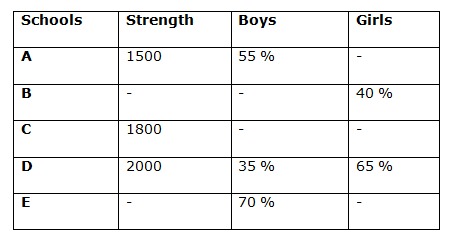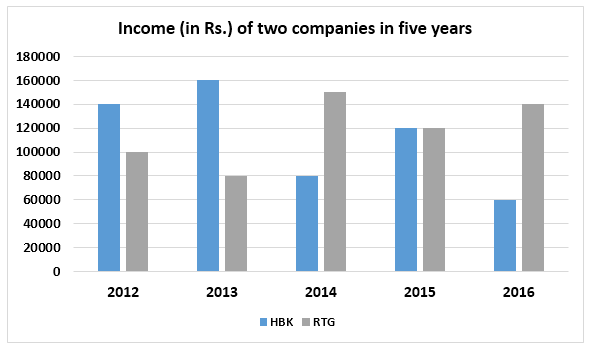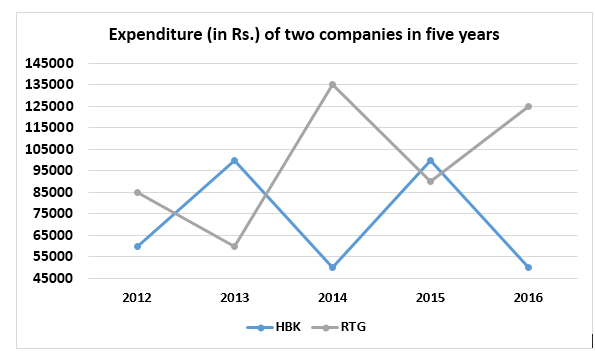# Quantitative Aptitude Questions (Data Interpretation) for NIACL Assistant Mains 2018 Day-177

Dear Readers, IBPS RRB is conducting Online Examination for the recruitment of Assistant. To enrich your preparation here we have providing new series of Data Interpretation – Quantitative Aptitude Questions. Candidates those who are appearing in NIACL Assistant  Mains Exams can practice these Quantitative Aptitude average questions daily and make your preparation effective.

[WpProQuiz 3376]

Click “Start Quiz” to attend these Questions and view Solutions

Directions (1 – 5): Study the following information carefully and answer the questions given below:

The following table represents strength of five different schools and the percentage of boys and girls in those schools.1) Number of girls in school A is approximately what percent of the number of girls in school D?

a) 36 %

b) 52 %

c) 44 %

d) 65 %

e) 28 %

2) If the strength of school B is 2500 and the strength of school E is 3500, number of girls of school E is what percent more than the number of girls of school B?

a) 25 %

b) 20 %

c) 5 %

d) 10 %

e) 15 %

3) If the ratio of number of boys and number of girls of school C is 5:1 respectively, then find the sum of boys in school C and number of boys of school D?

a) 2500

b) 1500

c) 1000

d) 2200

e) None of these

4) If the strength of school B is 2100, then find the respective ratio of number of girls of school B to that of school A?

a) 56: 45

b) 45: 56

c) 47: 45

d) 45: 46

e) None of these

5) The strength of schools A and C together is what percent more than the strength of school D?

a) 35%

b) 65%

c) 55%

d) 45%

e) None of these

Directions (6 – 10): Study the following information carefully and answer the questions given below:

The bar graph represents income of two companies in five years.

The line graph represents expenditure of two companies in five years.6) Percent profit earned by HBK in 2014 is what percent more than the percent profit earned by RTG in 2016?

a) 250%

b) 400%

c) 300%

d) 150%

e) None of these

7) Income of RTG in 2017 is 20% more than its income in 2015 and expenditure of the company in 2017 is 10% less than its expenditure in 2015. Find the respective ratio of percent profit earned by RTG in 2015 and 2017.

a) 3:4

b) 4:5

c) 3:7

d) 5:7

e) None of these

8) Find the difference between income of HBK in all the years together and expenditure of RTG in all the years together.

a) Rs.85000

b) Rs.65000

c) Rs.80000

d) Rs.75000

e) None of these

9) Profit percent of HBK in 2012 is approximately what percent of the profit percent of RTG in the same year?

a) 580%

b) 654%

c) 712%

d) 820%

e) 756%

10) Find the sum of percentage decrease in the income of HBK from 2012 to 2016 and percentage increase in the income of RTG from 2012 to 2016.

a) 89.99%

b) 78.88%

c) 67.67%

d) 97.14%

e) 83.33%

Direction (1-5) :

Percentage of girls in school A = 100 – 55 = 45%

Number of girls in school A = 1500*(45/100) = 675

Number of girls in school D = 2000*(65/100) = 1300

Required percentage = (675/1300)*100 = 51.92% = 52 %

Percentage of girls of school E = (100 – 70) = 30%

Number of girls of school E = 3500*(30/100) = 1050

Number of girls of school B = 2500*(40/100) = 1000

Required percentage = [(1050 – 1000)/1000]*100 = (50/1000)*100 = 5 %

Number of boys of school C = 1800*(5/6) = 1500

Number of boys of school D = 2000*(35/100) = 700

Required sum = 1500 + 700 = 2200

Number of girls of school B = 2100*(40/100) = 840

Number of girls of school A = 1500*(45/100) = 675

Required ratio = 840 : 675 = 56 : 45

Strength of schools A and C together = 1500 + 1800 = 3300

The strength of school D = 2000

Required percentage = [(3300 – 2000)/2000]*100 = 65%

Direction (6-10) :

We know that

% profit = (Income – Expenditure)/Expenditure x 100

Percent profit earned by HBK in 2014 = (80000 – 50000)/50000 x 100

= 30000/50000 x 100

= 60%

Percent profit earned by RTG in 2016 = (140000 – 125000)/125000 x 100

= 15000/125000 x 100

= 12%

Required percentage = (60 – 12)/12 x 100

= 48/12 x 100

= 400%

We know that

% profit = (Income – Expenditure)/Expenditure x 100

Percent profit earned by RTG in 2015 = (120000 – 90000)/90000 x 100

= 30000/90000 x 100

= 100/3%

Income of RTG in 2017 = 120000 x 120/100 = Rs.144000

Expenditure of RTG in 2017 = 90000 x 90/100 = Rs.81000

Percent profit earned by RTG in 2017 = (144000 – 81000)/81000 x 100

= 63000/81000 x 100

= 700/9%

Required ratio = 100/3: 700/9

= 3:7

Income of HBK in all the years together = 140000 + 160000 + 80000 + 120000 + 60000 = Rs.560000

Expenditure of RTG in all the years together = 85000 + 60000 + 135000 + 90000 + 125000 = Rs.495000

Required difference = 560000 – 495000 = Rs.65000

We know that

% profit = (Income – Expenditure)/Expenditure x 100

Profit percent of HBK in 2012 = (140000 – 60000)/60000 x 100

= 80000/60000 x 100

= 400/3%

Profit percent of RTG in 2012 = (100000 – 85000)/85000 x 100

= 15000/85000 x 100

= 300/17%

Required percentage = (400/3)/(300/17) x 100

= 755.55%

= 756% approx.

Percentage decrease in the income of HBK from 2012 to 2016

= (140000 – 60000)/140000 x 100

= 80000/140000 x 100

= 57.14%

Percentage increase in the income of RTG from 2012 to 2016

= (140000 – 100000)/100000 x 100

= 40000/100000 x 100

= 40%

Required sum = 57.14 + 40 = 97.14%

Daily Practice Test Schedule | Good Luck

 Topic Daily Publishing Time Daily News Papers & Editorials 8.00 AM Current Affairs Quiz 9.00 AM Quantitative Aptitude “20-20” 11.00 AM Vocabulary (Based on The Hindu) 12.00 PM General Awareness “20-20” 1.00 PM English Language “20-20” 2.00 PM Reasoning Puzzles & Seating 4.00 PM Daily Current Affairs Updates 5.00 PM Data Interpretation / Application Sums (Topic Wise) 6.00 PM Reasoning Ability “20-20” 7.00 PM English Language (New Pattern Questions) 8.00 PM